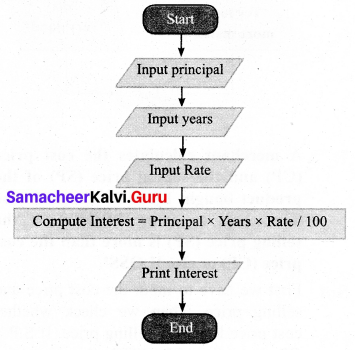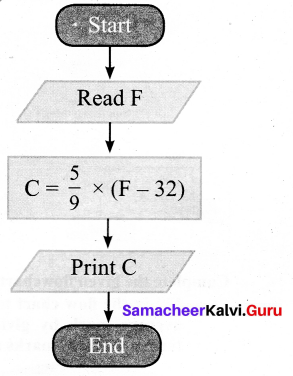# Samacheer Kalvi 7th Maths Solutions Term 3 Chapter 6 Information Processing Additional Questions

Students can Download Maths Chapter 6 Information Processing Questions and Answers, Notes Pdf, Samacheer Kalvi 7th Maths Book Solutions Guide Pdf helps you to revise the complete Tamilnadu State Board New Syllabus and score more marks in your examinations.

## Tamilnadu Samacheer Kalvi 7th Maths Solutions Term 3 Chapter 6 Information Processing Additional Questions

Exercise 6.1

Question 1.
Draw a flowchart to calculate simple interest for your bank deposit.
Solution:
Flow Chart:Algorithm:
(iii) Read rate of interest per year
(iv) Calculate the interest with formula Interest = Principal × Years × Rate/100
(v) Print InterestQuestion 2.
Draw a flowchart to convert the given Fahrenheit temperature to Celsius. Formula is C = $$\frac { 5 }{ 9 }$$ × (F – 32)
Solution:
Algorithm:
(ii) Calculate temperature in Celsius using formula C = $$\frac { 5 }{ 9 }$$ × (F – 32)